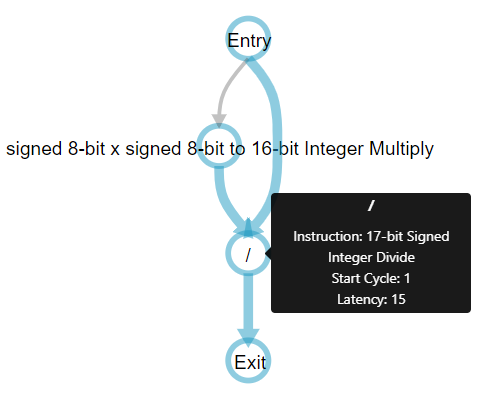ID 683349
Date 6/02/2023
Public

Give Feedback

## 8.1.2. Integer Promotion and ac_int Data Types

The rules of integer promotion when you use ac_int data types are different from the rules of integer promotion for standard C/C++ rules. Your component design should account for these differing rules.

Depending on the data type of the operands, integer promotion is carried out differently:
• Both operands are standard integer types (int, short, long, unsigned char, or signed char):

If both operands are of standard integer type (for example char or short) operations, integers can be promoted following the C/C++ standard. That is, the operation is carried out in the data type and size of the largest operand, but at least 32 bits. The expression returns the result in the larger data type.

• Both operands are ac_int data types:

If both operands are ac_int data types, operations are carried out in the smallest ac_int data type needed to contain all values. For example, the multiplication of two 8-bit ac_int values is carried out as an 16-bit operation. The expression returns the result in that type.

• One operand is a standard integer type and one operand is an ac_int type:

If the expression has one standard data type and one ac_int type, the rules for ac_int data type promotion apply. The resulting expression type is always an ac_int data type. For example, if you add a short data type and an ac_int<16> data type, the resulting data type is ac_int<17>.

### Data Width of Operations

C++ compilers typically automatically promote narrow integer types such as char and short to 32-bit types (int) for arithmetic operations such as addition, multiplication, division, and bit-shifts. To adhere to the C++ language specification and be consistent with other C++ compilers, the Intel® HLS Compiler might use larger operations than you might expect when dealing with native types.

If you need better control over the size of arithmetic operations, use the ac_int datatype.

Consider the following example:
component int singlestep_native_signed(char a, char b, char c)
{
return a * b / c;
}

The System Viewer (part of the High-Level Design Reports) shows that the divide operation becomes a 32-bit operation:Note: The Intel® HLS Compiler narrows operations automatically whenever it can, depending on the context of the operation. In this case, the compiler automatically narrowed the multiplication operations to 16 bits. To confirm the width of an operation, review your design in the System Viewer.
If you use ac_int data types for the parameters and the return data, the Intel® HLS Compiler makes the divide operation narrower.
#include <HLS/ac_int.h>
component int32 singlestep_acint_signed(int8 a, int8 b, int8 c)
{
return a * b / c;
}


With the component variables all defined as ac_int data types, the System Viewer now shows that the divide operation is reduced to being 17-bits wide:### Literals in Operations

In C/C++, literals are by default an int data type, so when you use a literal without any casting, the expression type is always at least 32 bits. For example, if you have code like following code snippet, the comparison is carried out in 32 bits:
ac_int<5, true> ap;
...
if (ap < 4) {
...

If the operands are signed differently and the unsigned type is at least as large as the signed type, the operation is carried out as an unsigned operations. Otherwise, the unsigned operand is converted to a signed operand.

For example, if you have code like the following snippet, the -1 value expands to a 32-bit negative number (0xffffffff) while the uint3 value is a positive 32-bit number 7 (0x00000007):
uint3 x = 7;
if (x != -1) {
// FAIL
}
To keep the compiler from expanding the literal to 32-bits, cast the literal to the same ac_int data type. For example:
uint3 x = 7;
if (x != (unit3)-1) {
// SUCCEED
}